# 0.展示PTA总分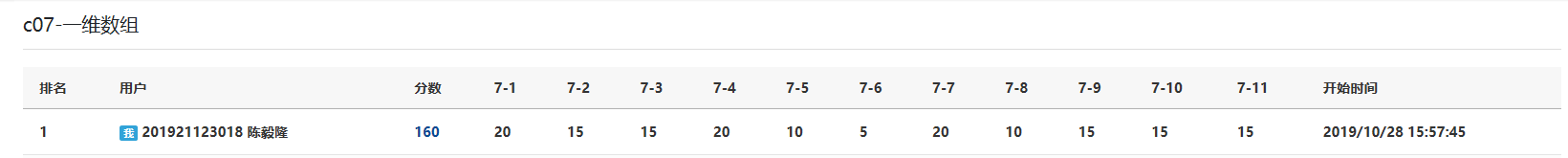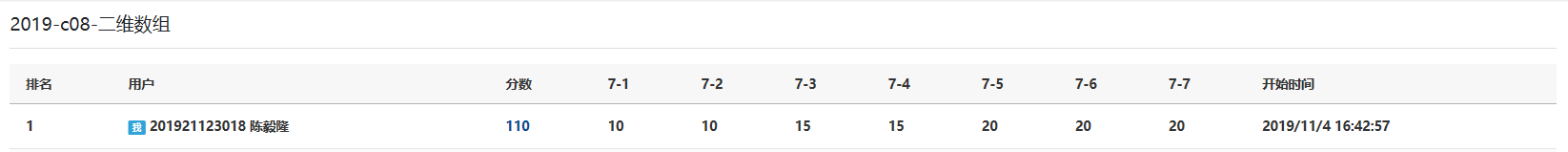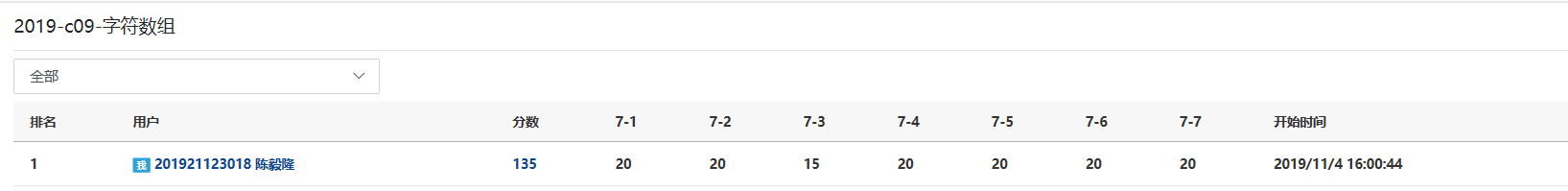# 1.本章学习总结

## 1.1 学习内容总结

• 数组中查找数据：
1、暴力查找法（历遍数组,找到所要的那个数据）：
利用下标 循环查找：比如a={1,2,3,4,5}中找到3这个数字
for(i=0;i<5;i++)
if(a[i]==3)
index=i;//于是记下3所在数组的下标，则a[index]就是所要找的数了
break；//结束循环


2、二分查找法（前提是在有序数列查找数据）:

int left = 0, right = n-1;//定义左界限left与右界限right

{
avg=(left+right)/2;
if（a[avg]==key），则输出avg；结束循环

}



• 数组中插入数据：
伪代码(比如一个a={1,2,3,4,5,7,8,9,10}中插入一个数x=6，按从小到大顺序)：
int i;

for(i=9;i>index;i--)
{
a[i]=a[i-1];
}
a[index]=x;


• 数组中删除数据：
• 比如有一个a={1,2,3,4,5},要在数组中删除3这个数据
1、伪代码(利用循环)：
for(i=0;i<5;i++)
if(a[i]==3)//找出3所在数组的下标
index=i;//记下下标
for(i=index;i<4;i++)
{
a[i]=a[i+1];
}//使a[index]赋值为下一位，然后执行循环使下一位赋值为下下一位；从而删除3这个数据，保留其他数据的数字的顺序
for(i=0;i<4;i++)
printf("%d"a[i]);
//在使用数组的时候，由于删除了一位数字，所以重新输出或者使用的时候，记得减少数组的一个长度，所以有i<4而不是i<5


2、伪代码（利用另一个数组）:

int i=0;j=0;
for(i=0;i<5;i++)
if(a[i]==3)//找出3所在数组的下标
index=i;//记下下标
while(j!=5)//这里如果数组长度为n,则将5改为n即可
{
if(i!=index)b[i++]=a[j++];
else {j++}
}


• 数组中目前学到排序方法
这里举例子并注释说明(有一个数组a[n],其中的数据要求从小到大排列)
1、冒泡法（把最大的沉下去）:
使用两层循环
for(i=1;i<n;i++)//外循环n-1次
{
for(j=0;j<n-1-i;j++)
{
判断a[j]>a[j+1]，是的话交换a[j]与a[j+1];
}//内循环结束后，将有一个最大值“沉”到未排序的最下面
}


2、选择法（先选择最大的，然后按照顺序排下来）：

for(i=1;i<n;i++)
{
for(j=0;j<n-i;j++)
{
如果a[MaxIndex]<a[j];则交换Max与j; //历遍数组，找出最大的值所在下标
}
交换a[MaxIndex]与a[n-i]; //将最大的元素与（未排序）下标最大的数组元素交换
}


• 数组做枚举用法:
• 7-2 IP地址转换:
伪代码：
fgets(a, 33, stdin);//输入一个32位长度的字符数组

for (i = 0; i < 32; i++)//历遍数组
{
sum = sum * 2 + a[i] - '0';//对每个数组元素都进行操作
if (i % 8 == 7)
{
if (i != 31)//输出对应的IP地址
printf("%d.", sum);
else printf("%d", sum);
sum = 0;
}
}


7-5 切分表达式——写个tokenizer吧：

 输入一串字符；
for (i = 0; a[i]; i++)
{
if在a位出现正负号，则不换行输出
if(a[i]是'.')，则不换行输出
if(a[i]是数字,a[i+1]是数字或'.')
则不换行输出
if(a[i]是'-'并且前一位是'('左括号)
则不换行输出
否则，其他均换行输出
}


• 哈希数组用法:
哈希数组的用法一般是定义2个数组，其中一个数组的数据是另外一个数组的下标，通过数据的导入改变另一个数组下标对应的数组数据；
在PTA上我找到了有4个例子：

7-5 有重复的数据I
（用数组的下标来判断另一个数组是否有重复数据）
PTA具体题目样例

7-9 调查电视节目受欢迎程度
（用数组存取被选择的节目的个数)
PTA具体题目样例

7-11 求整数序列中出现次数最多的数
(基本思路是用一个数组存取另一个数组数据对应下标的个数，最后找到次数最多的数，也就是另一个数组数据最大的数对应的下标)
PTA具体题目样例

7-4 删除重复字符：
（先判断是否有重复数据，然后删除对应的重复字符）
PTA具体题目样例

## 1.2 本章学习体会

• 学习感受：又过了2个周，突然觉得时间过得好快啊，从顺序结构到循环结构，再到函数，现在已经到了数组，学到的知识越来越多，但相对应的题目难道也越来越大了，自己也要更加的努力了。
这两周以来学习了数组，数组有3大类，一个是一维数组，第二个是二维数组，还有一个是字符数组，字符数组包括由简单的字符组成的数组，还有字符串数组，做数组的话感觉运用更灵活，思路也更复杂。二维数组的难度相较于一维数组的难度有成倍的增长。
• 这两周的代码量：884行

# 2.PTA实验作业

## 2.1 题目：7-7 螺旋方阵

### 2.1.1 伪代码：

for(k=0;k<5;k++)//控制螺旋赋值的次数
{
i = 0, j = 0;//重新赋值行、列
for(j = 0+k; j < n-k; j++)//控制赋值的数量
{
从左到右赋值方阵第一行；
}
for(i = 1+k; i <n-k; i++)//控制赋值的数量
{
从上到下赋值最后一列；
}
for(j = n - 2-k; j >= 0+k; j--)//控制赋值的数量
{
从右到左赋值最后一行；
}
for(i = n - 2-k; i >= 1+k; i--)//控制赋值的数量
{
从下到上赋值第一列；
}

}



### 2.1.2 代码截图：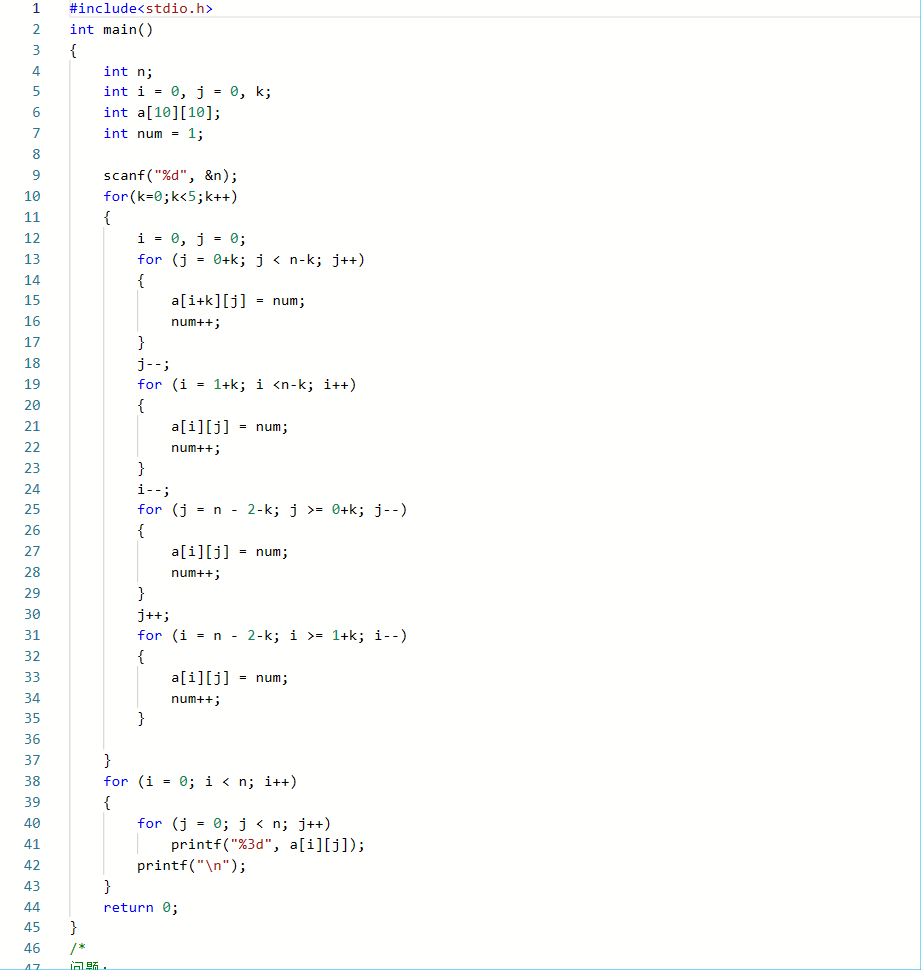### 2.1.3 造测试数据：

2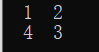刚好围成一圈
4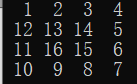普通的一个数据
9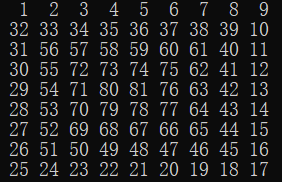临界最大的数据

### 2.1.4 PTA提交列表及说明：（在VS上已调试成功）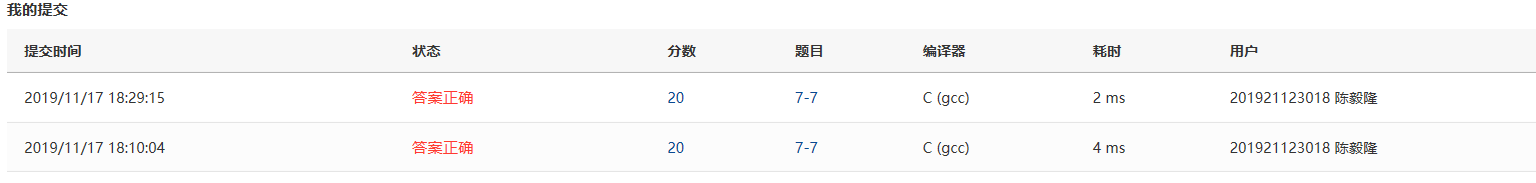### 提交列表说明：

• 问题1、螺旋方阵赋值时（内循环之间）出现了后一个for赋值把前面for最后一个拐角覆盖掉了，进行右移或左移后正常赋值
比如：
1 2 3 4
13 14 15 5
12 18 17 6
11 10 9 8
这里a原来应该被第2个for赋值为7的，结果却被第3个for重新赋值为8,还有外循环进行一次后的a也是出现了覆盖赋值的情况.
原来错误的循环：for (j = n - 1-k; j >= 0+k; j--)，改为for (j = n - 2-k; j >= 0+k; j--)，将j的初始值-1，使它赋值向左移了一位。
• 问题2、内循坏（自身）条件控制不好，也导致了覆盖赋值的情况
比如：
1 2 3 4
12 13 14 15
11 18 17 16
10 9 8 7
这里a原来应该为5,a原来应该为6，但是却被第二次外循环重新赋值为15、16；
于是将第1个内循坏由for (j = 0+k; j < n; j++)改为for (j = 0+k; j < n-k; j++)，改变循坏条件，使最后一个赋值变为有k控制的赋值。

## 2.2 题目：7-2 IP地址转换

### 2.2.1 伪代码：

输入32位数字字符；
for（i=0；i<32;i++）
{

sum重新初始化为0；
}


### 2.2.2 代码截图：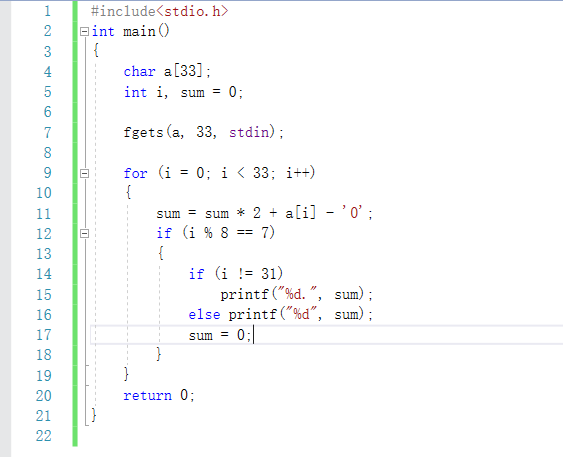### 2.2.3 造测试数据：

11001100100101000001010101110010 204.148.21.114 普通情况
00000000000000000000000000000000 0.0.0.0 全是0
11111111111111111111111111111111 255.255.255.255 全是1

### 2.2.4 PTA提交列表及说明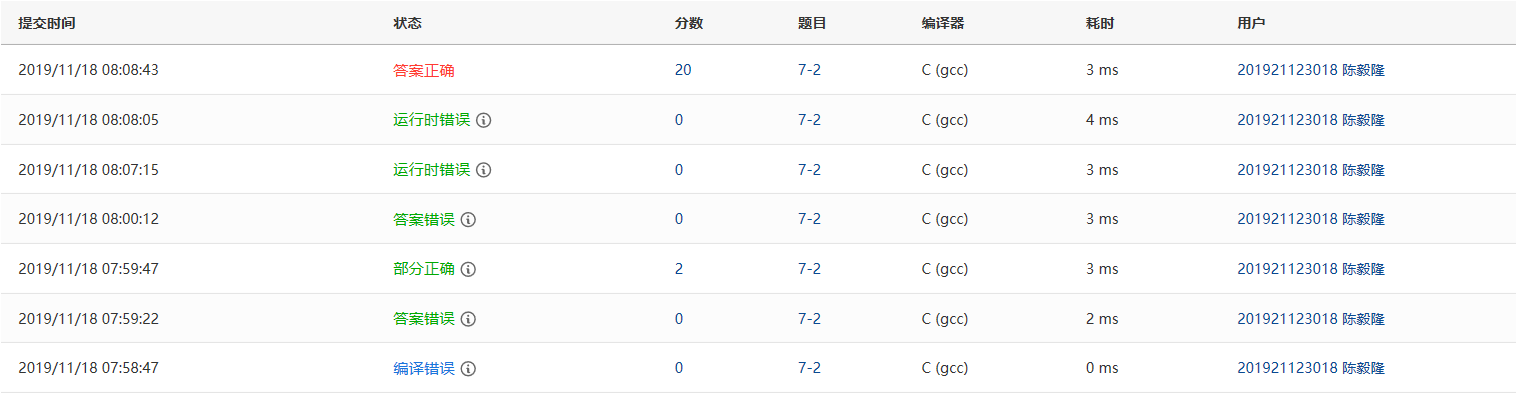### 提交列表说明：

1、编译错误：复制粘贴的时候结尾少了一个'}'，解决方法：补上}
2、部分正确：在每个sum赋值结束后没有重新s初始化为0；解决方法：在if（i%87）后面加sum=0，重新赋值。
3、答案错误：输出错误，原来是if（i%8
7）printf("%d."sum)；每个数字后面都紧跟一个'.'，答案错误；修改输出条件w：当i不等于31时，输出sum.，否则输出sum
4、运行时发生错误：一开始数组长度为32，用fgets无法储存32位数字字符，因为'\0'占了一个位置，导致最后一个数字被赋值位'\0'，无法赋值为正确的数字；解决方法：把数组a的长度扩大到33。并且在for循环中运用到了a.

## 2.3 题目名：7-3 字符串转换成十进制整数

### 2.3.1 伪代码：

char a,b;
int i，j=0；//j为b数组的下标
int flag=0;//用flag判断是否是负数，1为负数

end for;



### 2.3.2 代码截图: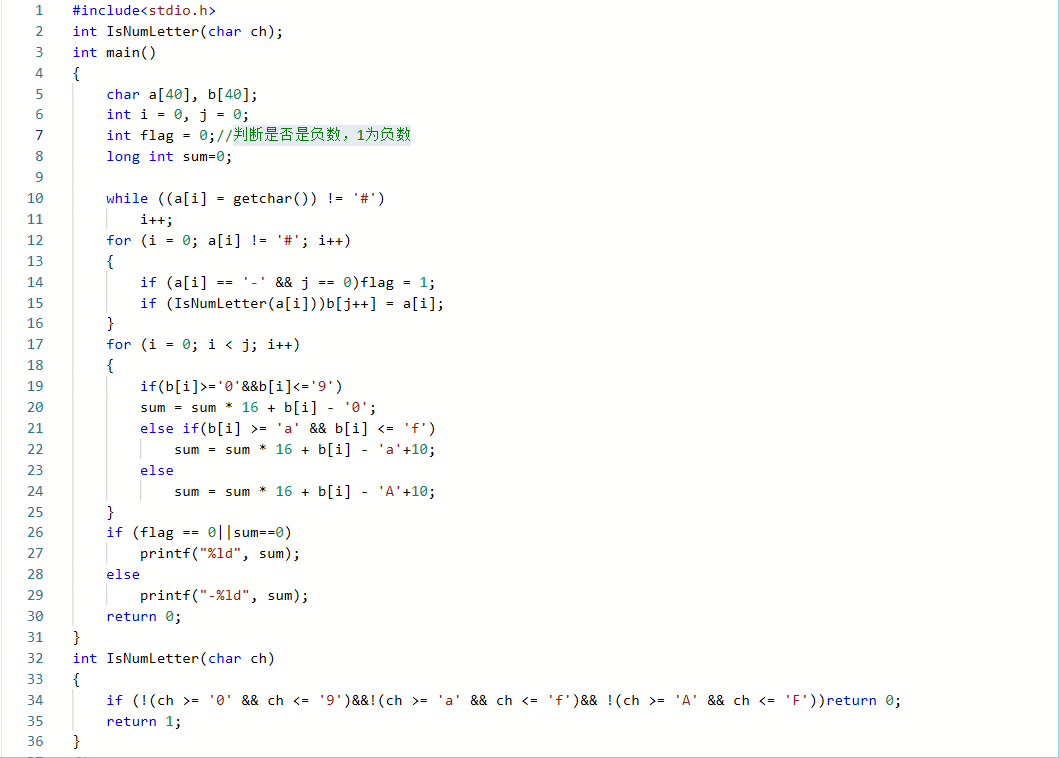### 2.3.3 造测试数据:

+-P-xf4+-1!# -3905 样例
-klx[o]i-m# n 全部过滤掉，不要输出-0
142af2# 1321714 字母数字都有

### 2.3.4 PTA提交列表及说明：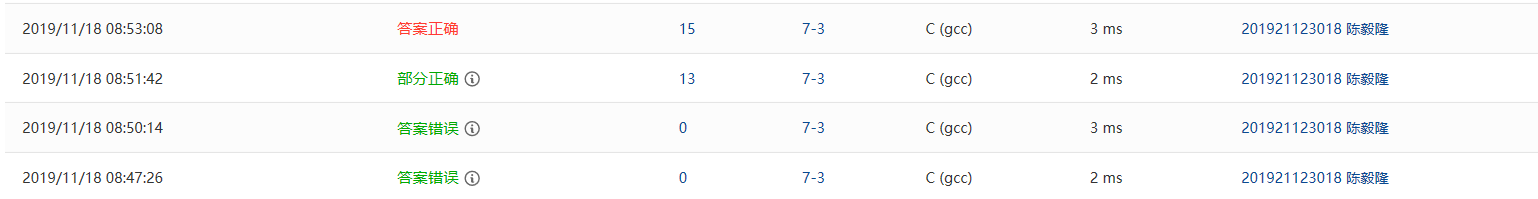### 提交列表说明：

1、答案错误：34行判断条件错误，不是(!(ch >= '0' && ch <= '9')||!(ch >= 'a' && ch <= 'f')|| !(ch >= 'A' && ch <= 'F'))，

2、答案错误：22行、24行运算错误；原本是sum = sum * 16 + b[i] - 'a'；sum = sum * 16 + b[i] - 'A'

3、部分正确：17行循环条件错误：for (i = 0; i < j-1; i++)，应改为for (i = 0; i < j; i++)
4、部分正确：全部过滤掉，不要输出-0测试点没过；解决方法：在输出的时候c增加判断条件，如果sum==0则输出sum就行了，而不是输出-sum。

# 3.阅读代码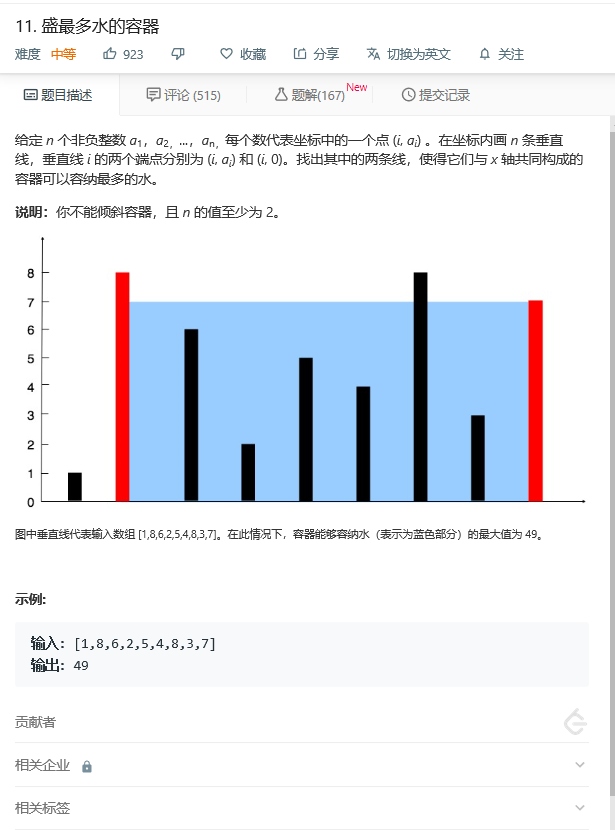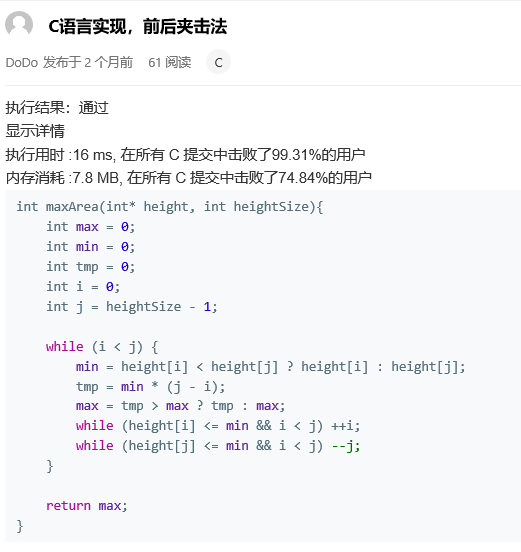while（i<j）//从左界限到右界限历遍
min = height[i] < height[j] ? height[i] : height[j];//选择出数组两个数据数值其中的较小值（木桶效应：面积由最小的木板决定）

tmp = min * (j - i);//计算面积
max = tmp > max ? tmp : max;//又是一个三目运算符?: 选择出面积大的那一位
while (height[i] <= min && i < j) ++i;
while (height[j] <= min && i < j) --j;

posted @ 2019-11-17 22:33  陈毅隆  阅读(716)  评论(0编辑  收藏  举报